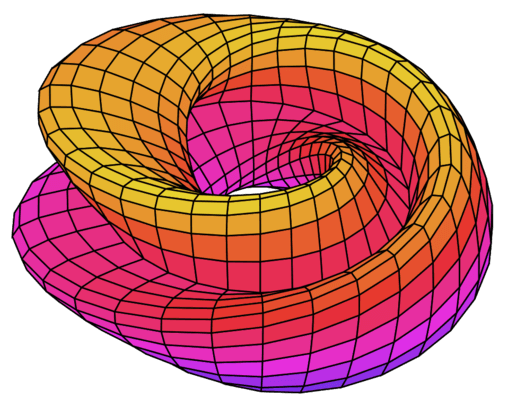Categories

# Pythagorean Triple, ISI Subjective Entrance 2018, Problem 7

## Understand the problem

Let $a,b,c \in \mathbb{N}$ be such that
$a^2+b^2=c^2$ and $c-b=1$.
Prove that
(i) $a$ is odd,
(ii) $b$ is divisible by 4,
(iii) $a^b+b^a$ is divisible by $c$.

##### Source of the problem
I.S.I. (Indian Statistical Institute) B.Stat/B.Math Entrance Examination 2018. Subjective Problem no. 7.
Number Theory

8 out of 10

##### Suggested Book

Elementary Number Theory‘ by David M. Burton
‘Challenge and Thrill of Pre-College Mathematics’ by V,Krishnamurthy, C.R.Pranesachar, ect.

Do you really need a hint? Try it first!

$a^2+b^2=c^2=(b+1)^2=b^2+2b+1$ $\Rightarrow a^2=2b+1$ Which implies $a^2$ is odd integer. $\Rightarrow a$ is also an odd integer =$2k+1$ (say).

$(2k+1)^2+b^2=c^2$ $\Rightarrow 4k^2+4k+1+b^2=b^2+2b+1$ $\Rightarrow b=2k(k+1)$. Now $k(k+1)$ is always even =$2l$ (say). Therefore, $b=4l$, i.e. $b$ is divisible by 4.

$a^b+b^a=a^{4l}+b^{2k+1}=(a^2)^{2l}+(c-1)^{2k+1}$ =$(2b+1)^{2l}+(c-1)^{2k+1}$ =$(2c-1)^{2l}+(c-1)^{2k+1}.$

Now $2l$ is even , therefore , $(2c-1)^{2l}$ is of the form : $2cp+1$ where $p \in \mathbb{N}$. And $2k+1$ is odd , therefore $(c-1)^{2k+1}$ is of the form : $cq-1$, where $q \in \mathbb{N}$. Therefore $a^b+b^a=(2cp+1)+(cq-1)=c\cdot (2p+q)$. $\Rightarrow c|a^b+b^a$.

## Connected Program at Cheenta# I.S.I. & C.M.I. Entrance Program

Indian Statistical Institute and Chennai Mathematical Institute offer challenging bachelor’s program for gifted students. These courses are B.Stat and B.Math program in I.S.I., B.Sc. Math in C.M.I.

The entrances to these programs are far more challenging than usual engineering entrances. Cheenta offers an intense, problem-driven program for these two entrances.

## How to use Vectors and Carpet Theorem in Geometry 1?

Here is a video solution for a Problem based on using Vectors and Carpet Theorem in Geometry 1? This problem is helpful for Math Olympiad, ISI & CMI Entrance, and other math contests. Watch and Learn! Here goes the question… Given ABCD is a quadrilateral and P and...

## Mahalanobis National Statistics Competition

Mahalanobis National Statistics Competition = MNStatC organized by Cheenta Statistics Department with exciting cash prizes. What is MNStatC? Mahalanobis National Statistics Competition (MNStatC) is a national level statistics competition, aimed at undergraduate...

## Carpet Strategy in Geometry | Watch and Learn

Here is a video solution for a Problem based on Carpet Strategy in Geometry. This problem is helpful for Math Olympiad, ISI & CMI Entrance, and other math contests. Watch and Learn! Here goes the question… Suppose ABCD is a square and X is a point on BC such that...

## Bijection Principle Problem | ISI Entrance TOMATO Obj 22

Here is a video solution for a Problem based on Bijection Principle. This is an Objective question 22 from TOMATO for ISI Entrance. Watch and Learn! Here goes the question… Given that: x+y+z=10, where x, y and z are natural numbers. How many such solutions are...

## What is the Area of Quadrilateral? | AMC 12 2018 | Problem 13

Here is a video solution for a Problem based on finding the area of a quadrilateral. This question is from American Mathematics Competition, AMC 12, 2018. Watch and Learn! Here goes the question… Connect the centroids of the four triangles in a square. Can you find...

## Solving Weird Equations using Inequality | TOMATO Problem 78

Here is a video solution for ISI Entrance Number Theory Problems based on solving weird equations using Inequality. Watch and Learn! Here goes the question… Solve: 2 \cos ^{2}\left(x^{3}+x\right)=2^{x}+2^{-x} We will recommend you to try the problem yourself. Done?...

## AM-GM Inequality Problem | ISI Entrance

Here is a video solution for ISI Entrance Number Theory Problems based on AM-GM Inequality Problem. Watch and Learn! Here goes the question… a, b, c, d are positive real numbers. Prove that: (1+a)(1+b)(1+c)(1+d) <= 16. We will recommend you to try the problem...

## Sum of 8 fourth powers | ISI Entrance Problem

Here is a video solution for ISI Entrance Number Theory Problems based on Sum of 8 fourth powers. Watch and Learn! Can you show that the sum of 8 fourth powers of integers never adds up to 1993? How can you solve this fourth-degree diophantine equation? Let’s...

## ISI MStat Entrance 2020 Problems and Solutions

Problems and Solutions of ISI MStat Entrance 2020 of Indian Statistical Institute.

## ISI Entrance 2020 Problems and Solutions – B.Stat & B.Math

Problems and Solutions of ISI BStat and BMath Entrance 2020 of Indian Statistical Institute.

This site uses Akismet to reduce spam. Learn how your comment data is processed.